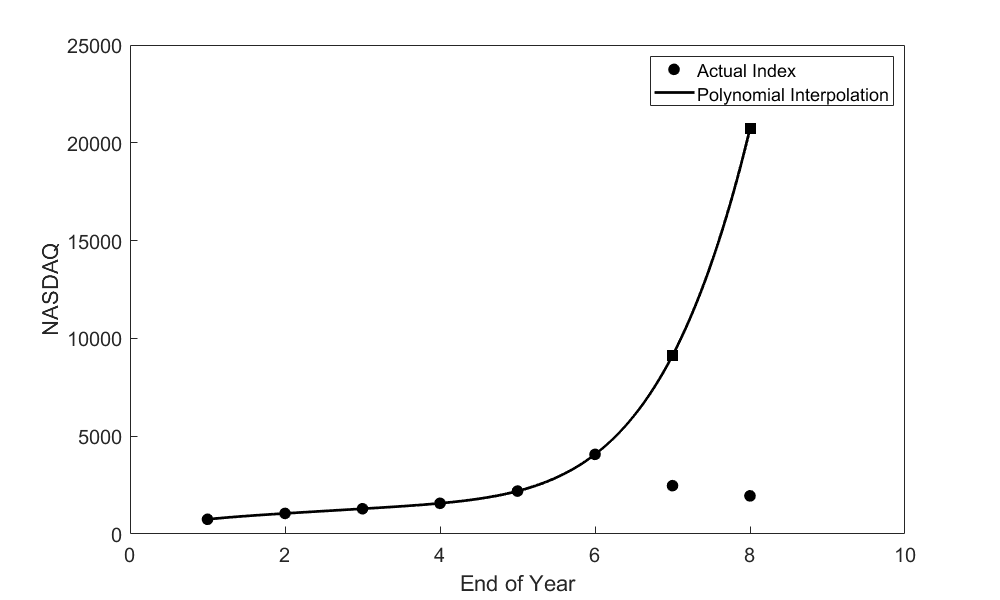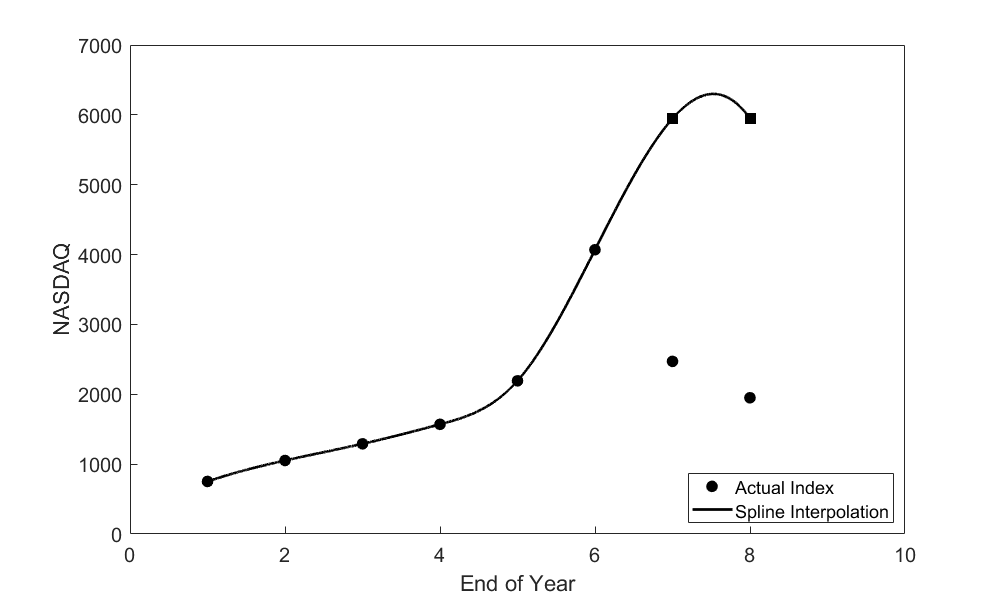# Chapter 05.06: Extrapolation is a Bad Idea

## Learning Objectives

After successful completion of this lesson, you should be able to:

1) enumerate why using extrapolation can be a bad idea.

2) show through an example why extrapolation can be a bad idea.

## Description

This conversation illustrates the pitfall of using extrapolation.

(Due to certain reasons, this student wishes to remain anonymous.)

This takes place in Summer Session B – July 2001

Student: “Hey, Dr. Kaw! Look at this cool new cell phone I just got!”

Kaw: “That’s nice. It better not ring in my class or it’s mine.”

Student: “What would you think about getting stock in this company?”

Kaw: “What company is that?”

Student: “WorldCom! They’re the world’s leading global data and internet company.”

Kaw: “So?”

Student: “They’ve just closed the deal today to merge with Intermedia Communications, based right here in Tampa!”

Kaw: “Yeah, and …?”

Student: “The stock’s booming! It’s at \$14.11 per share and promised to go only one way—up! We’ll be millionaires if we invest now!”

Kaw: “You might not want to assume their stock will keep rising … besides, I’m skeptical of their success. I don’t want you putting yourself in financial ‘jeopardy!’ over some silly extrapolation. Take a look at these NASDAQ composite numbers (Table 1).”

Student: “That’s only up to two years ago …”

Kaw: “That’s right. Looking at this data, don’t you think you should’ve invested back then?”

Student: “Well, didn’t the composite drop after that?”

Kaw: “Right again, but look what you would’ve hoped for if you had depended on that trend continuing (Figure 1).”

Student: “So you’re saying that …?”

Kaw: “You should seldom depend on extrapolation as a source of approximation! Just take a look at how wrong you would have been (Table 2).”

Table 1. End of year NASDAQ composite data

End of year NASDAQ
$$1$$ $$751.96$$
$$2$$ $$1052.13$$
$$3$$ $$1291.03$$
$$4$$ $$1570.35$$
$$5$$ $$2192.69$$
$$6$$ $$4069.31$$

Note: The range of years in Table 1 are actually between 1994 (Year 1) and 1999 (Year 9). Numbers start from 1 to avoid round-off errors and near singularities in matrix calculations.Figure 1 Data from 1994 to 1999 extrapolated to yield results for 2000 and 2001 using polynomial extrapolation.

Table 2 Absolute relative true error of polynomial interpolation.

End of Year Actual

Fifth order

polynomial interpolation

Absolute relative

true error
$$2000$$ $$2471$$ $$9128$$ $$269.47 \%$$
$$2001$$ $$1950$$ $$20720$$ $$962.36 \%$$

Student: “Now wait a sec! I wouldn’t have been quite that wrong. What if I had used cubic splines instead of a fifth-order interpolant?”

Kaw: “Let’s find out.”Figure 2 Data from 1994 to 1999 extrapolated to yield results for 2000 and 2001 using cubic spline interpolation.

Table 3 Absolute relative true error of cubic spline interpolation

End of Year Actual

Cubic spline

interpolation

Absolute relative

true error
$$2000$$ $$2471$$ $$5945.9$$ $$140.63 \%$$
$$2001$$ $$1950$$ $$5947.4$$ $$204.99 \%$$

Student: “There you go. That didn’t take so long (Figure 2 and Table 3).”

Kaw: “Well, let’s think about what this data means. If you had gone ahead and invested, thinking your projected yield would follow the spline, you would have only been 205% (Table 3) wrong, as opposed to being 962% (Table 2) wrong by following the polynomial. That’s not so bad, is it?”

Student: “Okay, you’ve got a point. Maybe I’ll hold off on being an investor and just use the cell phone.”

Kaw: “You’ve got a point, too—you’re brighter than you look … that is if you turn off the phone before coming to class.”

* * * * *

<One year later … July 2002>

Student: “Hey, Dr. Kaw! Whatcha got for me today?”

Kaw: “The Computational Methods students just took their interpolation test today, so here you go. <hands stack of tests to student> Time to grade them!”

Student: <Grunt!> “That’s a lot of paper! Boy, interpolation … learned that a while ago.”

Kaw: “You haven’t forgotten my lesson to you about not extrapolating, have you?”

Student: “Of course not! Haven’t you seen the news? WorldCom just closed down 93% from 83¢ on June 25 to 6¢ per share! They’ve had to recalculate their earnings, so your skepticism really must’ve spread. Did you have an”in” on what was going on?”

Kaw: “Oh, of course not. I’m just an ignorant numerical methods professor.”